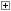•About ADP
•Download and Install
•Command-line syntax
•Grammer
•Library alphabetical list
•Library by category
•Error messages
•Samples
•History and Roadmap
•License and Support and Other
•Blog

# Tutorials (example of code）

##### Hello Wold
・program code（helloworld.p）
```,printn("Hello World.");
```
・example of the execution
```D:\sample>adp helloworld.p
Hello World.
```
ADP command loads and compiles the program. Then, ADP evaluates goal clauses, executes the program.

This sample, hellowrld.p, is evaluating predicate of 'printn'.
'printn' is one of the embedded predicate, like library, print arguments to the console and line feed.

##### Example of arithmetic operations
・program code（expression.p）
```,\$x=10 ,\$y=20  ,printn( \$x + \$y ); # add
,\$x=30 ,\$y=40  ,printn( \$x - \$y ); # sub
,\$x=50 ,\$y=60  ,printn( \$x * \$y ); # multiply
,\$x=70 ,\$y=80  ,printn( \$x / \$y ); # divide
,\$x=90 ,\$y=100 ,printn( \$x % \$y ); # modulo
```
・example of the execution
```D:\sample>adp expression.p
30
-10
3000
0
90
```
ADP supports arithmetic operations.

##### About executing goal clause (Each term has the executing result)
Each term in a goal clause has the boolean value of the executing result.
If the executing result is true, then ADP will execute next term.
If the executing result is false, then ADP will backtrack, will be described in the next section, instead of execute next term.

・program code（condition.p）
```,\$x = 10 ,\$y = 20 ,\$x < \$y ,printn("\$x is smaller than \$y."); # -- (1)
,\$x = 10 ,\$y = 20 ,\$x > \$y ,printn("\$x is bigger than \$y."); # -- (2)
```

・example of the execution
```D:\sample>adp condition.p
\$x is smaller than \$y.
```

Predicate 'printn' at (1) is executed, showing '\$x is smaller than \$y'.
But predicate 'printn' at (2) is not executed, because the expression right before the predicate '\$x > \$y' returns false. \$x is 10, \$y is 20, and \$x(10) > \$y(20) is false.
ADP backtracked after executing the expression.